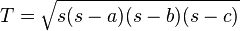# If we want maths teachers, we must reward them – Editorials – The Independent

I agree with the premise that we must demonstrate that we value maths teachers, in order to attract the best. Money isn’t always the answer, though it is a part of the issue.

# Trigonometry – Herons formulawhere s is the semiperimeter of the triangle:# Zequals: the new symbol that will make us all better at sums | Rob Eastaway | Science | guardian.co.uk

## Euclid (Book 1, Definition 22)

“Of quadrilateral figures, a square is that which is both equilateral and right-angled; an oblong that which is right-angled but not equilateral; a rhombus that which is equilateral but not right-angled; and a rhomboid that which has opposite sides and angles equal to one another but is neither equilateral nor right angled. And let quadrilaterals other than these be called trapezia.”

RECTANGLE is  a four-sided shape with four right angles, and includes:

SQUARE which has four right angles AND four equal sides

OBLONG which has four right angles BUT two pairs of equal sides (not four equal sides)

RHOMBUS is a four-sided shape with four equal sides BUT no right-angles

RHOMBOID is a four-sided shape with two pairs of equal sides, and opposite angles are equal (also a PARALLELOGRAM)

TRAPEZIUM is a  four-sided shape with one pair of parallel sides (called a TRAPEZOID in America!)

TRAPEZOID is a four-sided shape with no parallel sides (called a TRAPEZIUM in America!)